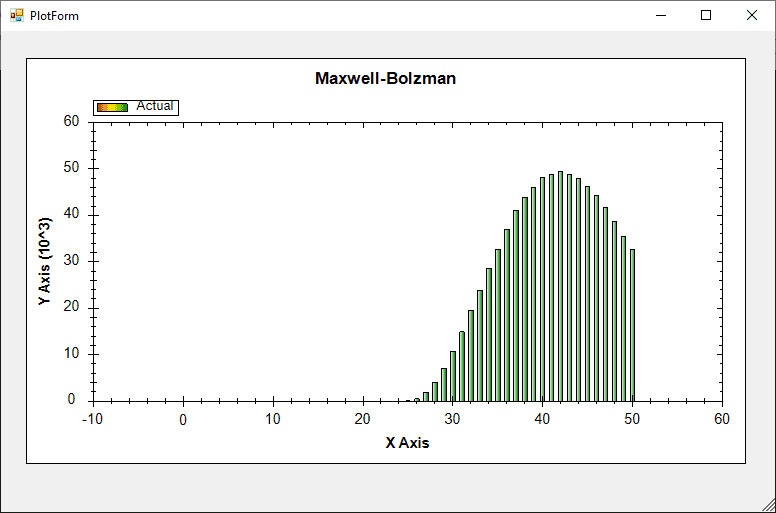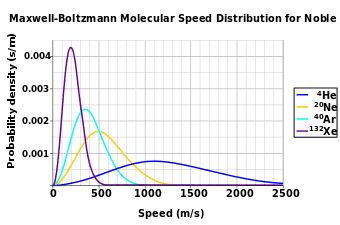# How can I draw a histogram from Maxwell's distribution using C#?

• Engineering
Gold Member
Homework Statement:
I need to create a histogram of Maxwell-Boltzman's distribution.
Relevant Equations:
https://en.wikipedia.org/wiki/Maxwell%E2%80%93Boltzmann_distribution#Distribution_for_the_speed
I have written the following source code:

Maxwell-Bolzman:
using System;

public class CommonDistributions
{
public static double Uniform(Random random)
{
return random.NextDouble();
}

static double Gaussian(Random random)
{
return Math.Sqrt(-2 * Math.Log(Uniform(random))) * Math.Cos(2 * Math.PI * Uniform(random));
}
public static double Gaussian(Random random, double mu, double sigma)
{
return sigma * Gaussian(random) + mu;
}
}

public class MaxwellBolzman
{
static double KB = 1.38064852e-23;

static double MaxwellVariance(double mass, double temperature)
{
return Math.Sqrt(KB * temperature / mass);
}

static double MaxwellComponent(Random random, double mass, double temperature)
{
double mu = 0.0;
double sigma = MaxwellVariance(mass, temperature);

return CommonDistributions.Gaussian(random, mu, sigma);
}
public static double Maxwell(Random random, double mass, double temperature)
{
double one = MaxwellComponent(random, mass, temperature);
double two = MaxwellComponent(random, mass, temperature);
double thr = MaxwellComponent(random, mass, temperature);

return Math.Sqrt(one * one + two * two + thr * thr);
}
}

public static class Normalization
{
public static int Normalize(double n_bins, double mu, double sigma, double gaussian)
{
var z = (gaussian - mu) / sigma;

if (z > 3 || z < -3)
{
return -1;
}
else
{
return (int)((z + 3) * n_bins / 6d);
}
}
}

class Program
{
static void Main(string[] args)
{
const double N = 1000000;
int time = (int)N;
int binsCount = 51;

Random rand = new Random();
int[] bins = new int[binsCount];

double mass = 14 * 1.67e-27;
double T = 300;

for (int i = 0; i < time; i++)
{
double gauss = MaxwellBolzman.Next(rand, mass, T);

int index = Normalization.Normalize(binsCount, mass, T, gauss);

if (index >= 0)
{
bins[index]++;
}
}

PointPairList list = new PointPairList();
for (int i = 0; i < bins.Length; i++)
{
}

PlotForm form = new PlotForm("Maxwell-Bolzman");
form.AxisChange();
form.ShowDialog();
}
}

It gives the following output:But, according to this link, the graph should look like the following: